# OliNo

Renewable Energy

## KLV Ledverlichting – KLV-MAT5-847A test.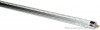presents herewith a T5 ledtube working on 24 V DC that emits a very cold white light.

This article shows the measurement results. Many parameters are also found in the Eulumdat file.

See this overview for a comparison with other light bulbs.

### Summary measurement data

parameter meas. result remark
Color temperature 8741 K very cold white
Luminous intensity Iv 302.0 Cd Measured straight underneath the lamp.
Illuminance modulation index 0 % Measured with a light sensor looking at the lamp (angle not defined). Is a measure for the amount of flickering.
Beam angle 121 deg 120 deg is the beam angle for the C0-C180-plane (perpendicular to the length direction of the lamp) and 121 deg is the beam angle for the C90-C270 plane, which is along the length direction of the lamp.
Power Factor NaN The tests were done with a DC power supply. This results in no blind power and as a result the power factor is always 1.0 but not relevant to mention.
THD NaN % Total Harmonic Distortion, is not present as a DC voltage was used to power the lamp so a DC current resulted which has no THD.
Luminous flux 941 lm
Luminous efficacy 91 lm/W Be aware that a DC power supply has been used. The found efficacy with this measurement is excluding the lpower supply that normally is needed to convert the grid voltage (230 V AC) to the used DC voltage. By excluding the consumption of the power supply the efficay found here is higher than it would be when the power supply had been included.
EU-label classification A The energy class, from A (more efficient) to G (least efficient).
CRI_Ra 78 Color Rendering Index.
Coordinates chromaticity diagram x=0.2881 en y=0.2998
Fitting FL-tube This lamp is connected to a 24 V DC voltage.
PAR-value 3.0 uMol/s/m2 The number of photons seen by an average plant when it is lit by the light of this light bulb. Value valid at 1 m distance from light bulb.
PAR-photon efficacy 0.9 uMol/s/We The total emitted number of photons by this light, divided by its consumption in W. It indicates a kind of efficacy in generating photons.
S/P ratio 2.4 This factor indicates the amount of times more efficient the light of this light bulb is perceived under scotopic circumstances (low environmental light level).
L x W x H external dimensions 845 mm x 15 mm x 15 mm External dimensions of the lamp.
L x W luminous area 823 mm x 9 mm Dimensions of the luminous area (used in Eulumdat file). This is the surface of the pcb on which the leds are mounted.
General remarks The ambient temperature during the whole set of illuminance measurements was 22.9 – 24.4 deg C.

The temperature of the housing gets maximally about 18 degrees hotter than ambient temperature.

Warm up effect: during the warm up time the illuminance increases about 8 % and the consumed power increases about 8 %.

Voltage dependency: There is a constant dependency of the illuminance when the power voltage varies between 22 – 26 V DC.
There is a constant dependency of the consumed power when the power voltage varies between 22 – 26 V DC.

At the end of the article an additional photo.

Measurement report (PDF)Eulumdat fileRight click on icon and save the file.

### Overview tableThe overview table is explained on the OliNo website.

Please note that this overview table makes use of calculations, use this data with care as explained on the OliNo site. E (lux) values are not accurate, when within 5 x 823 mm (maximal luminous size, eventually diagonally measured)= 4115 mm. Within this distance from the lamp, the measured lux values will be less than the computed values in this overview as the measurements are then within the near field of the lamp.

### EU Energy label classification

With the measurement results of the luminous flux and the consumed power the classification on energy efficacy of this lamp is calculated. This information is requested in the EU for certain household lamps, see also the OliNo site that explains for which lamps it is requested, how the label looks like and what information it needs to contain.

Herewith the labels for this lamp in color and black and white.EU energy label of this lamp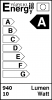Label in black and white.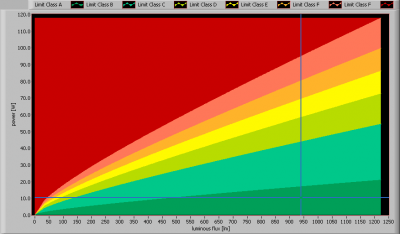The lamp’s performance in the lumen-Watt field, with the energy efficacy fields indicated.

### Eulumdat light diagram

This light diagram below comes from the program Qlumedit, that extracts these diagrams from an Eulumdat file. This light diagram is explained on the OliNo site.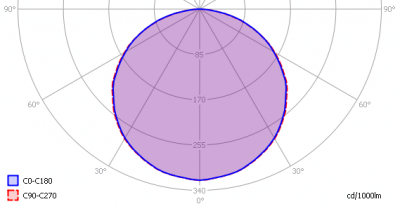The light diagram giving the radiation pattern.

The light diagram indicates the beam in the C0-C180 plane (perpendicular to the length direction of the lamp) and in the plane perpendicular to that, the C90-C270 plane (along the length direction of the lamp).

### Illuminance Ev at 1 m distance, or luminous intensity Iv

Herewith the plot of the averaged luminous intensity Iv as a function of the inclination angle with the light bulb.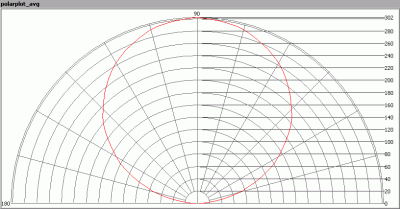The radiation pattern of the light bulb.

This radiation pattern is the average of the light output of the light diagram given earlier. Also, in this graph the luminous intensity is given in Cd.

These averaged values are used (later) to compute the lumen output.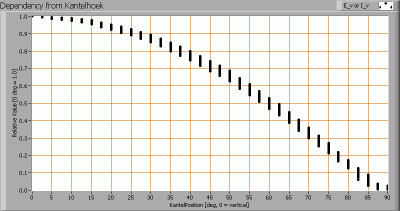Intensity data of every measured turn angle at each inclination angle.

This plot shows per inclination angle the intensity measurement results for each turn angle at that inclination angle. There normally are differences in illuminance values for different turn angles. However for further calculations the averaged values will be used.

When using the average values per inclination angle, the beam angle can be computed, being 120 deg for the C0-C180 plane and 121 deg for the C90-C270 plane.

### Luminous flux

With the averaged illuminance data at 1 m distance, taken from the graph showing the averaged radiation pattern, it is possible to compute the luminous flux.

The result of this computation for this light spot is a luminous flux of 941 lm.

### Luminous efficacy

The luminous flux being 941 lm, and the consumed power of the lamp being 10.3 Watt, results in a luminous efficacy of 91 lm/Watt.

### Electrical properties

The power factor is NaN. The tests were done with a DC power supply. This results in no blind power and as a result the power factor is always 1.0 but not relevant to mention.

 Lamp voltage 24.0 V Lamp current 0.430 A Power P 10.3 W Apparent power S 10.3 VA Power factor NaN

### Temperature measurements lamp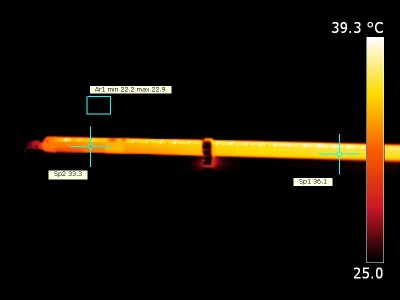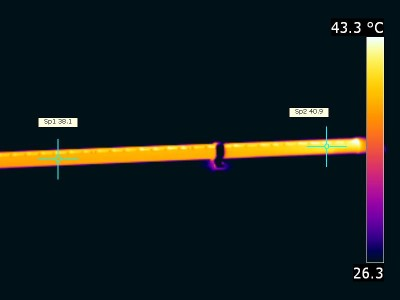The emissivity of the alu material is 0.87..

 status lamp > 2 hours on ambient temperature 22.5 deg C reflected background temperature 22.5 deg C camera Flir T335 emissivity 0.87 measurement distance 1.5 m IFOVgeometric 0.136 mm per 0.1 m distance NETD (thermal sensitivity) 50 mK

### Color temperature and Spectral power distributionThe spectral power distribution of this light bulb, energies on y-axis valid at 1 m distance.

The measured color temperature is 8741 K which is very cold white.

This color temperature is measured straight underneath the light bulb. Below a graph showing the color temperature for different inclination angles.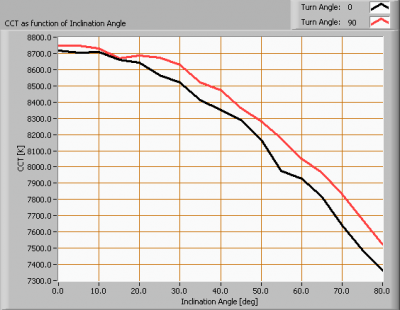Color temperature as a function of inclination angle.

The color temperature is given for inclination angles up to 80 deg. Beyond that value the illuminance is so low (< 5 lux) that it has not been used for color determination of the light.

For the C0-C180 plane: the beam angle of 120 deg is equivalent to 59.8 deg inclination angle,which is the area where most of the light falls within. The maximum variation of color temperature in this inclination area is about 8 %.

For the C90-C270 plane: the beam angle of 121 deg is equivalent to 60.3 deg inclination angle,which is the area where most of the light falls within. The maximum variation of color temperature in this inclination area is about 9 %.

### PAR value and PAR spectrum

To make a statement how well the light of this light bulb is for growing plants, the PAR-area needs to be determined. See the explanation about PAR on the OliNo website how this all is determined and the explanation of the graph.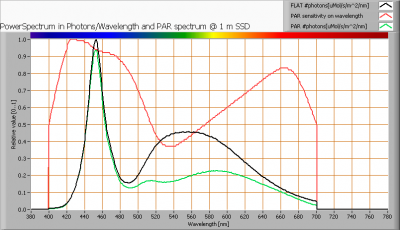The photon spectrum, then the sensitivity curve and as result the final PAR spectrum of the light of this light bulb

parameter value unit
PAR-number 3.0 uMol/s/m^2
PAR-photon current 9.3 uMol/s
PAR-photon efficacy 0.9 uMol/s/W

The PAR efficiency is 67 % (valid for the PAR wave length range of 400 – 700 nm). This is the maximum percentage of the total of photons in the light that is effectively used by the average plant (since the plant might not take 100 % of the photons at the frequency where its relative sensitivity is 100 %).

### S/P ratio

The S/P ratio and measurement is explained on the OliNo website. Here the results are given.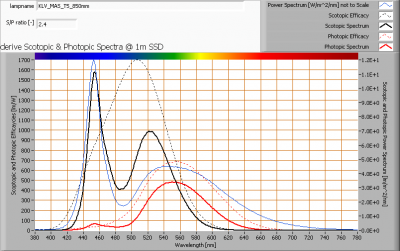The power spectrum, sensitivity curves and resulting scotopic and photopic spectra (spectra energy content defined at 1 m distance).

The S/P ratio of the light coming from this lamp is 2.4.

More info on S/P ratio can be found on the OliNo website.

### Chromaticity diagramThe chromaticity space and the position of the lamp’s color coordinates in it.

The point of the light in this diagram is outside the area indicated with class A. This area indicates an area for signal lamps, see also the article on signal lamps and color areas on the OliNo website.

The color coordinates are x=0.2881 and y=0.2998.

### Color Rendering Index (CRI) or also Ra

Herewith the image showing the CRI as well as how well different colors are represented (rendered). The higher the number, the better the resemblance with the color when a black body radiator would have been used (the sun, or an incandescent lamp). Practical information and also some critics about the CRI can be found on the OliNo website.

Each color has an index Rx, and the first 8 indexes (R1 .. R8) are averaged to compute the Ra which is equivalent to the CRI.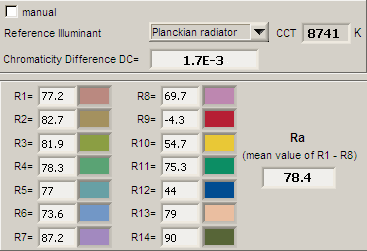CRI of the light of this lightbulb.

This value of 78 indicates how well the light of this lamp can render well a set of reference colors, this in comparison with the light of a reference source (for color temperatures < 5000K a black radiator is used as reference and for color temperatures > 5000K the sun or the light outside during the day).

The value of 78 is marginally smaller than the value of 80 that is considered as a minimum for working areas in general.

Note: the chromaticity difference is 0.0017 and indicates the distance to the Planckian Locus. There is no norm yet that states what the max deviation from white light is allowed to be. A reference with signal lights as a reference is given in the chromaticity diagram.

### Voltage dependency

The dependency of a number of lamp parameters on the lamp voltage is determined. For this, the lamp voltage has been varied and its effect on the following light bulb parameters measured: illuminance E_v [lx], the lamppower P [W] and the luminous efficacy [lm/W] (this latter is estimated here by dividing the found E_v value by P).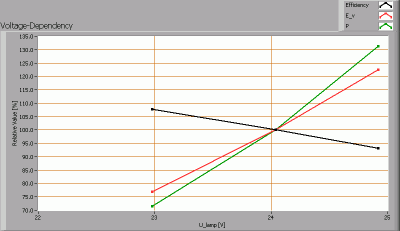Lamp voltage dependencies of certain light bulb parameters

There is a constant dependency of the illuminance when the power voltage varies between 22 – 26 V DC.
There is a constant dependency of the consumed power when the power voltage varies between 22 – 26 V DC.

When the voltage varies abruptly with + or – 0.5 V DC then this results in a variation of the illuminance of maximally 12.7 %. This difference in illuminance is possibly visible (when it occurs abruptly).

### Warm up effects

After switch on of a cold lamp, the effect of heating up of the lamp is measured on illuminance E_v [lx], the lamppower P [W] and the luminous efficacy [lm/W].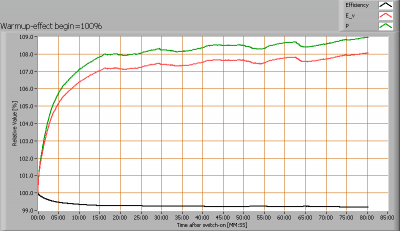Effect of warming up on different light bulb parameters. In the first graph the 100 % level is put at begin, and in the last graph the 100 % level is put at the end.

The warmup time is about 20 minutes during which the illuminance increases with 8 % and the consumed power increases with 8 %.

### Measure of flickering

An analysis is done on the measure of flickering of the light output by this light bulb. See the article on flickering on OliNo site for more information.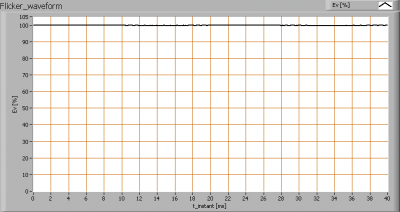The measure of fast illuminance variation of the light of the light bulb

parameter value unit
Flicker frequency 52.7 Hz
Illuminance modulation index 0 %

The illuminance modulation index is computed as: (max_Ev – min_Ev) / (max_Ev + min_Ev).